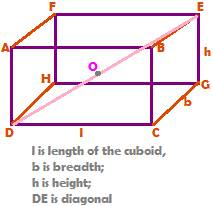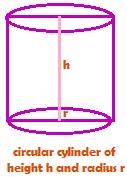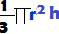## Surface Area and Volume of Solids

1. Cube

Let side of a cube be s.

Then, commit to memory the following formulas in the cube

Lateral surface area = 4s2

Total surface area = 6s2

Volume = s3

Diagonal = s × √3

2. CuboidLet l, b and h denote the length, breadth and height of a Cuboid respectively.

Then, commit to memory the following formulas in the cuboid

Lateral surface area = 2 (lh + bh)                   {lh = l × h, bh = b × h}

Total surface area = 2 (lb + lh + bh)

Volume = l × b × h

Diagonal of a Cuboid is

3. Right Circular cylinderLet r and h be radius and height of a right circular cylinder. Then,

Commit to memory the following formulas in a right circular cylinder

Curved surface area = 2rh

Total surface are = 2r2 + 2rh = 2r(r + h)

Volume = r2h

4. Circular cone

Let r be radius; h, the vertical height; and l, the slant height in a right circular cone.

Then,

Curved surface area = rl

Total surface area = rl + r2

Volume =5. Sphere

Let ‘r’ denote radius of a sphere. Then,

Total surface area of the sphere = 4r2

Volume of the sphere =

6. Hemi sphere

Let ‘r’ denote radius of a hemi sphere. Then,

Curved surface area = 4r2

Total surface area = 3r2

Volume =